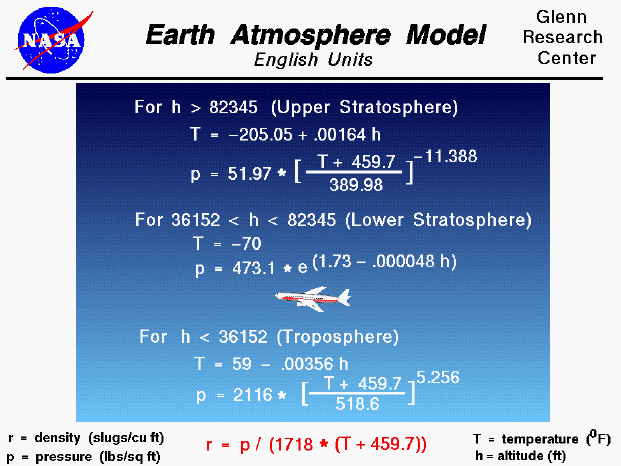A text only version of this slide is available which gives all of the flow equations.

The Earth's atmosphere is an extremely thin sheet of air extending from the surface of the Earth to the edge of space. If the Earth were the size of a basketball, a tightly held pillowcase would represent the thickness of the atmosphere. Gravity holds the atmosphere to the Earth's surface. And within the atmosphere, very complex chemical, thermodynamic, and fluid dynamics effects occur. The atmosphere is not uniform; fluid properties are constantly changing with time and place. (We call this change the weather.)

These variations extend upward from the surface of the Earth. The sun heats the surface of the Earth, and some of this heat goes into heating the air near the surface. The heated air is then diffused or convected up through the atmosphere. Thus the air temperature will be highest near the surface and decreases as altitude increases. The speed of sound depends on the temperature and also decreases with increasing altitude. The pressure of the air can be related to the weight of the air over a given location. As we increase altitude through the atmosphere, there will be some air below us and some air above us. But there will always be less air above us than was present at a lower altitude. Therefore, air pressure decreases as we increase altitude. The air density depends on both the temperature and the pressure through the equation of state and also decreases with increasing altitude.

Aerodynamic forces directly depend on the air density. To help aircraft designers, it is useful to define a standard atmosphere model. (There are actually many different models available--a standard or average day, a hot day, a cold day, a tropical day. And the models are updated every few years to include the latest atmospheric data.) The model was developed from atmospheric measurements that were averaged and curve fit to produce the given equations. The model assumes that the pressure and temperature change only with altitude. The particular model shown here was developed in the early sixties, and the curve fits are given for English units. These curve fits are also available in metric units. The model has three parts: one for the lower atmosphere, in which temperature decreases with altitude; one for the lower stratosphere, in which the temperature is constant; and one for the upper stratosphere, in which the temperature increases with altitude. In each part, the pressure and temperature are curve fit; and the density is derived from the equation of state. This is the model used in the FoilSim and EngineSim programs.

An interactive Java applet for this slide is also available. With the applet, you can change altitude and see the effects on pressure and temperature.Guided Tours

Beginner's Guide to Aerodynamics
Beginner's Guide to Propulsion
Beginner's Guide to Model Rockets
Beginner's Guide to Kites
Beginner's Guide to Aeronautics